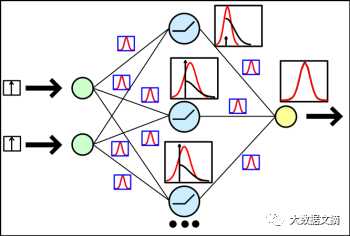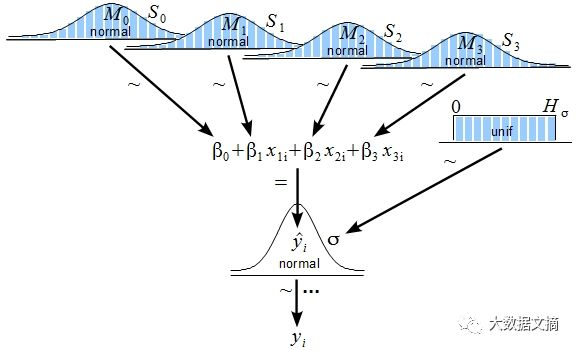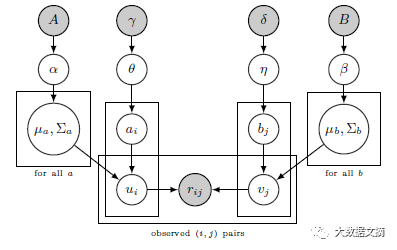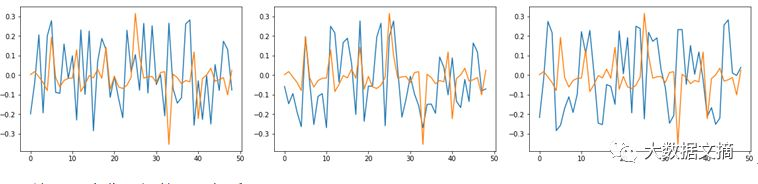# 手把手：基于概率编程Pyro的金融预测，让正则化结果更有趣！

2018-04-12 文摘菌 大数据文摘Hi！又见面啦。去年我推出了几篇基于神经网络的金融预测教程，我认为有些结果还是蛮有趣的，值得应用在实际交易中。

• 模式识别和机器学习：

http://www.springer.com/us/book/9780387310732

• 为黑客设计的贝叶斯方法：

• PyMC3：

https://github.com/pymc-devs/pymc3

• Edward：

http://edwardlib.org/

• Pyro：

http://pyro.ai/https://bitinfocharts.com/Pyro官方教程：

http://pyro.ai/examples/bayesian_regression.html

class RegressionModel(nn.Module):
def __init__(self, p):
super(RegressionModel, self).__init__()
self.linear
= nn.Linear(p, 1)
def forward(self, x):
# x * w + b
return self.linear(x)

def model(data):
# Create unit normal priors over the parameters
mu
= Variable(torch.zeros(1, p)).type_as(data)
sigma = Variable(torch.ones(1, p)).type_as(data)
bias_mu = Variable(torch.zeros(1)).type_as(data)
bias_sigma = Variable(torch.ones(1)).type_as(data)
w_prior, b_prior = Normal(mu, sigma), Normal(bias_mu, bias_sigma)
priors = {‘linear.weight’: w_prior, ‘linear.bias’: b_prior}
lifted_module = pyro.random_module(“module”, regression_model, priors)
lifted_reg_model = lifted_module()
with pyro.iarange(“map”, N, subsample=data):
x_data = data[:, :-1]
y_data = data[:, -1]
# run the regressor forward conditioned on inputs
prediction_mean = lifted_reg_model(x_data).squeeze()
pyro.sample(“obs”,
Normal(prediction_mean, Variable(torch.ones(data.size(0))).type_as(data)),
obs=y_data.squeeze())

def guide(data):
w_mu
w_log_sig = Variable(0.1 * torch.ones(1, p).type_as(data.data), requires_grad=True)
b_log_sig = Variable(0.1 * torch.ones(1).type_as(data.data), requires_grad=True)
mw_param = pyro.param(“guide_mean_weight”, w_mu)
sw_param = softplus(pyro.param(“guide_log_sigma_weight”, w_log_sig))
mb_param = pyro.param(“guide_mean_bias”, b_mu)
sb_param = softplus(pyro.param(“guide_log_sigma_bias”, b_log_sig))
w_dist = Normal(mw_param, sw_param)
b_dist = Normal(mb_param, sb_param)
dists = {‘linear.weight’: w_dist, ‘linear.bias’: b_dist}
lifted_module = pyro.random_module(“module”, regression_model, dists)
return lifted_module()

``（~Normal(0, 0.1)）``

for j in range(3000):
epoch_loss
= 0.0
perm = torch.randperm(N)
# shuffle data
data = data[perm]
# get indices of each batch
all_batches = get_batch_indices(N, 64)
for ix, batch_start in enumerate(all_batches[:-1]):
batch_end = all_batches[ix + 1]
batch_data = data[batch_start: batch_end]
epoch_loss += svi.step(batch_data)

preds = []
for i in range(100):
sampled_reg_model = guide(X_test)
pred = sampled_reg_model(X_test).data.numpy().flatten()
preds.append(pred)30天的贝叶斯模型预测

def get_model(input_size):
main_input
= Input(shape=(input_size, ), name=‘main_input’)
x = Dense(25, activation=‘linear’)(main_input)
output = Dense(1, activation = “linear”, name = “out”)(x)
final_model = Model(inputs=[main_input], outputs=[output])
return final_model

model = get_model(len(X_train))
history = model.fit(X_train, Y_train,
epochs = 100,
batch_size = 64,
verbose=1,
validation_data=(X_test, Y_test),
callbacks=[reduce_lr, checkpointer],
shuffle=True)30天的Keras神经网络预测

class Net(torch.nn.Module):
def __init__(self, n_feature, n_hidden):
super(Net, self).__init__()
self.hidden
= torch.nn.Linear(n_feature, n_hidden)   # hidden layer
self.predict = torch.nn.Linear(n_hidden, 1)   # output layer
def forward(self, x):
x = self.hidden(x)
x = self.predict(x)
return x

priors = {‘hidden.weight’: w_prior,
‘hidden.bias’: b_prior,
‘predict.weight’: w_prior2,
‘predict.bias’: b_prior2}

dists = {‘hidden.weight’: w_dist,
‘hidden.bias’: b_dist,
‘predict.weight’: w_dist2,
‘predict.bias’: b_dist2}30天的Pyro神经网络预测

for name in pyro.get_param_store().get_all_param_names():
print name, pyro.param(name).data.numpy()

import tensorflow as tf
sess = tf.Session()
with sess.as_default():
tf.global_variables_initializer().run()
dense_weights, out_weights = None, None
with sess.as_default():
for layer in model.layers:
if len(layer.weights) > 0:
weights = layer.get_weights()
if ‘dense’ in layer.name:
dense_weights = layer.weights.eval()
if ‘out’ in layer.name:
out_weights = layer.weights.eval()https://medium.com/@alexrachnog/financial-forecasting-with-probabilistic-programming-and-pyro-db68ab1a1dba